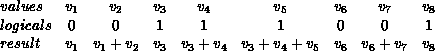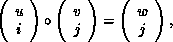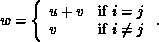## 4.11.1. Example using MPI_SCANUp: Scan Next: Correctness Previous: Scan

Example

This example uses a user-defined operation to produce a segmented scan. A segmented scan takes, as input, a set of values and a set of logicals, and the logicals delineate the various segments of the scan. For example:The operator that produces this effect is,where,Note that this is a non-commutative operator. C code that implements it is given below.

```typedef struct {
double val;
int log;
} SegScanPair;

/* the user-defined function
*/
void segScan( SegScanPair *in, SegScanPair *inout, int *len,
MPI_Datatype *dptr )
{
int i;
SegScanPair c;

for (i=0; i< *len; ++i) {
if ( in->log == inout->log )
c.val = in->val + inout->val;
else
c.val = inout->val;
c.log = inout->log;
*inout = c;
in++; inout++;
}
}
```
Note that the inout argument to the user-defined function corresponds to the right-hand operand of the operator. When using this operator, we must be careful to specify that it is non-commutative, as in the following.

```int i,base;
MPI_Op       myOp;
MPI_Datatype type = {MPI_DOUBLE, MPI_INT};
MPI_Aint     disp;
int          blocklen = { 1, 1};
MPI_Datatype sspair;

/* explain to MPI how type SegScanPair is defined
*/
base = disp;
for (i=0; i<2; ++i) disp[i] -= base;
MPI_Type_struct( 2, blocklen, disp, type, &sspair );
MPI_Type_commit( &sspair );
/* create the segmented-scan user-op
*/
MPI_Op_create( segScan, False, &myOp );
...
MPI_Scan( a, answer, 1, sspair, myOp, root, comm );
```Up: Scan Next: Correctness Previous: Scan